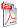Issue archive

https://doi.org/10.15255/KUI.2021.048
Published: Kem. Ind. 71 (3-4) (2022) 185–199
Paper reference number: KUI-48/2021
Paper type: Original scientific paper
Download paper:PDFKinetic Parameters Estimation and Model Evaluation for the Aerobic Bioconversion Process of the Organic Fraction of Municipal Solid Waste

E. Papraćanin

##### Abstract

Aerobic bioconversion is very interesting process for the mathematical modelling and optimisation from the aspect of improving and accelerating the process, which is reflected in a higher organic matter decomposition rate. The aim of this research was to estimate the kinetic parameters in a dynamic model that described aerobic biodegradation of organic fraction of municipal solid waste (OFMSW) with various additions, from an engineering aspect. The presented mathematical model based on heat and mass transfer phenomena is a dynamic model for the gas-liquid-solid system. For the optimization and process simulation, three experiments were performed in three geometrically identical reactor systems at the same time, with different initial composition of composting mixtures. Data obtained experimentally from one reactor were used to optimize kinetic parameters of the model, and data from the other two reactors were used to verify and evaluate the dynamic model. Detailed statistical analysis showed narrow values of 95 % confidence interval for estimated parameters, indicating the appropriate accuracy of parameter estimation. Better data agreement was achieved in the reactor with a smaller ratio of OFMSW and additives, and the most accurately predicted dynamic state variable was the mass of organic matter. Parameter sensitivity analysis showed that the most sensitive parameters were reaction order n and activation energy E. Overall, the application of this model with optimised kinetic parameters of the aerobic biodegradation process represents a tool to predict the most important process variables (SD = 0.3378).

##### Keywords

mathematical modelling, kinetic parameters, model evaluation, sensitivity analysis, aerobic biodegradation, OFMSW, waste yeast, kieselguhr